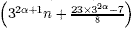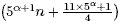Congruences for Bipartitions with Odd Parts Distinct

William Y.C. Chen and Bernard L.S. Lin

 Abstract:  Hirschhorn and Sellers studied arithmetic properties of the number of partitions with odd parts distinct. In another direction, Hammond and Lewis investigated arithmetic properties of the number of bipartitions. In this paper, we consider the number of bipartitions with odd parts distinct. Let this number be denoted by pod-2(n). We obtain two Ramanujan type identities for pod-2(n), which imply that pod-2(2n+1) is even and pod-2(3n+2) is divisible by 3. Furthermore, we show that for any α ≥ 1 and n ≥ 0, pod-2is a multiple of 3 and pod-2is divisible by 5. We also find combinatorial interpretations for the two congruences modulo 2 and 3.   AMS Classification:  05A17, 11P83   Keywords:  partition, bipartition, congruence, birank   Download:   pdf

Return